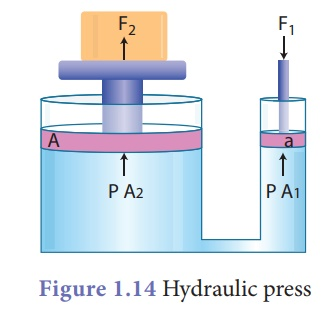Home | | Science 9th std | Pascal's Law

# Pascal's Law

Pascal's principle is named after Blaise Pascal (1623-1662), a French mathematician and physicist. The law states that the external pressure applied on an incompressible liquid is transmitted uniformly throughout the liquid.

Pascal's Law

Pascal's principle is named after Blaise Pascal (1623-1662), a French mathematician and physicist. The law states that the external pressure applied on an incompressible liquid is transmitted uniformly throughout the liquid. Pascal’s law can be demonstrated with the help of the glass vessel having holes all over its surface. Fill it with water. Push the piston. The water rushes out of the holes in the vessel with the same pressure. The force applied on the piston exerts pressure on water. This pressure is transmitted equally throughout the liquid in all directions (Fig. 1.13). This principle is applied in various machines used in our daily life.## Hydraulic press

Pascal's law became the basis for one of the important machines ever developed, the hydraulic press. It consists of two cylinders of different cross-sectional areas as shown in Figure 1.14. They are fitted with pistons of cross-sectional areas “a” and “A”. The object to be compressed is placed over the piston of large cross-sectional area A.The force F1 is applied on the piston of small cross-sectional area a. The pressure P produced by small piston is transmitted equally to large piston and a force F2 acts on A which is much larger than F1. Pressure on piston of small area ‘a’ is given by,

P = F1/A1              ………(1)

Applying Pascal’s law, the pressure on large piston of area A will be the same as that on small piston. Therefore, P = F2/A2         ……………..(2)

Comparing equations (1) and (2),we get  F1/A1 = F2/A2 or F2 = F1  x A2/A1

Since, the ratio A2/A1 is greater than 1, theforce F2 that acts on the larger piston is greater than the force F1 acting on the smaller piston. Hydraulic systems working in this way are known as force multipliers.

### Example 1.5

A hydraulic system is used to lift a 2000 kg vehicle in an auto garage. If the vehicle sits on a piston of area 0.5 m2, and a force is applied to a piston of area 0.03 m2, what is the minimum force that must be applied to lift the vehicle?

### Given:

Area covered by the vehicle on the piston A1 = 0.5 m2

Weight of the vehicle, F1 = 2000 kg × 9.8 m s–2

Area on which force F2 is applied, A2 = 0.03 m2

### Solution:

P1 = P2 ; F1/A1 = F2/A2 and F2 = [ F1/A1] .A2 ;

F2 = (2000 × 9.8) [0.03/0.5] = 1176 N

### Example 1.6

Two syringes are connected together as shown in the diagram below.

A force of 20 N is applied to the piston in syringe A.

(a) Calculate the pressure that the piston in syringe A exerts on the oil.

(b) Calculate the force needed to just prevent the piston in syringe B from moving out.### Solution:

(a) A force of 20 N is applied to the piston in syringe A.

The pressure that the piston in syringe A exerts on the oil,

P = F/A = 20N/0.5cm2 = 40 N cm-2

(b)  P = F/A . So F = PA

The force needed to just prevent the piston in syringe B from moving out,

F = 40 N cm–2 × 5 cm2 = 200 N

Tags : Hydraulic press | Fluids , 9th Science : Fluids
Study Material, Lecturing Notes, Assignment, Reference, Wiki description explanation, brief detail
9th Science : Fluids : Pascal's Law | Hydraulic press | Fluids